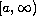Electron. J. Diff. Eqns., Vol. 2006(2006), No. 145, pp. 1-8.

### Oscillation for forced second-order nonlinear dynamic equations on time scales Mugen Huang, Weizhen Feng

Abstract:
By means of Riccati transformation techniques, we present oscillation criteria for forced second-order nonlinear dynamic equations on time scales. These results are based on the information on a sequence of subintervals ofonly, rather than on the whole half-line.

Submitted August 18, 2006. Published November 26, 2006.
Math Subject Classifications: 34K11, 39A10, 39A99, 34C10, 39A11.
Key Words: Forced oscillation; dynamic equations; time scales.

Show me the PDF file (207K), TEX file, and other files for this article.Mugen Huang School of Mathematical Sciences South China Normal University, Guangzhou 510631, China email: huangmugen@sohu.com Weizhen Feng School of Mathematical Sciences South China Normal University, Guangzhou 510631, China email: wsy@scnu.edu.cn